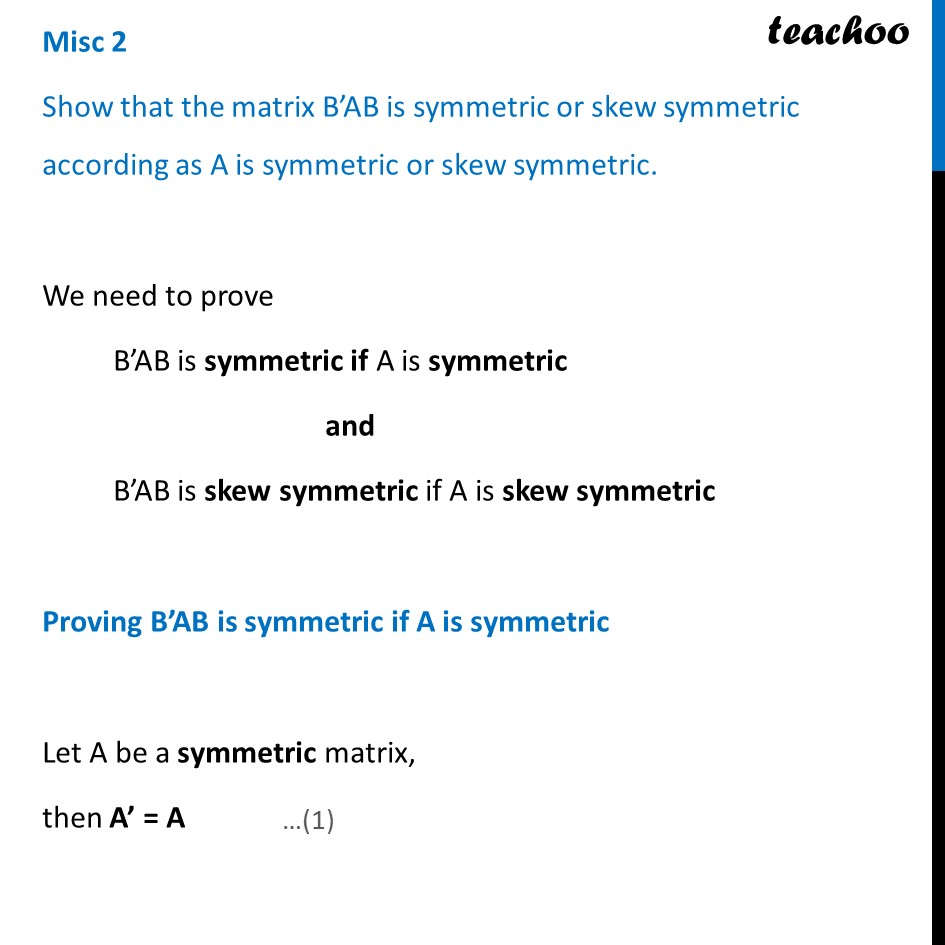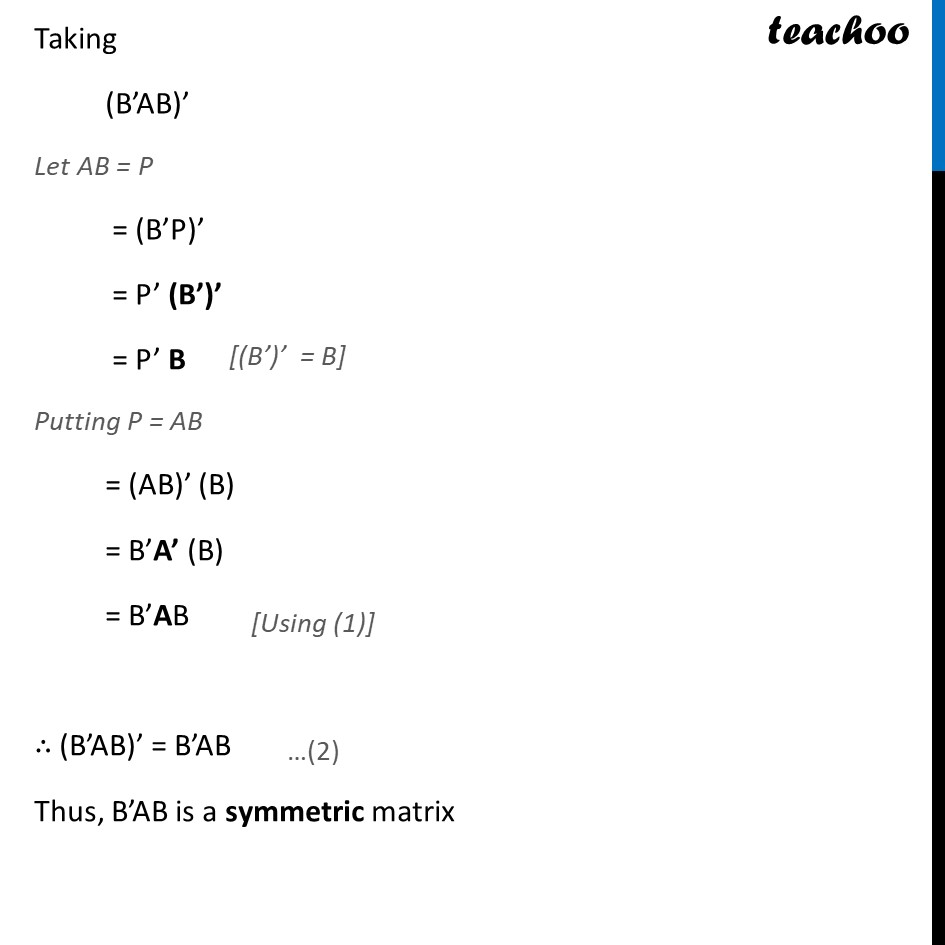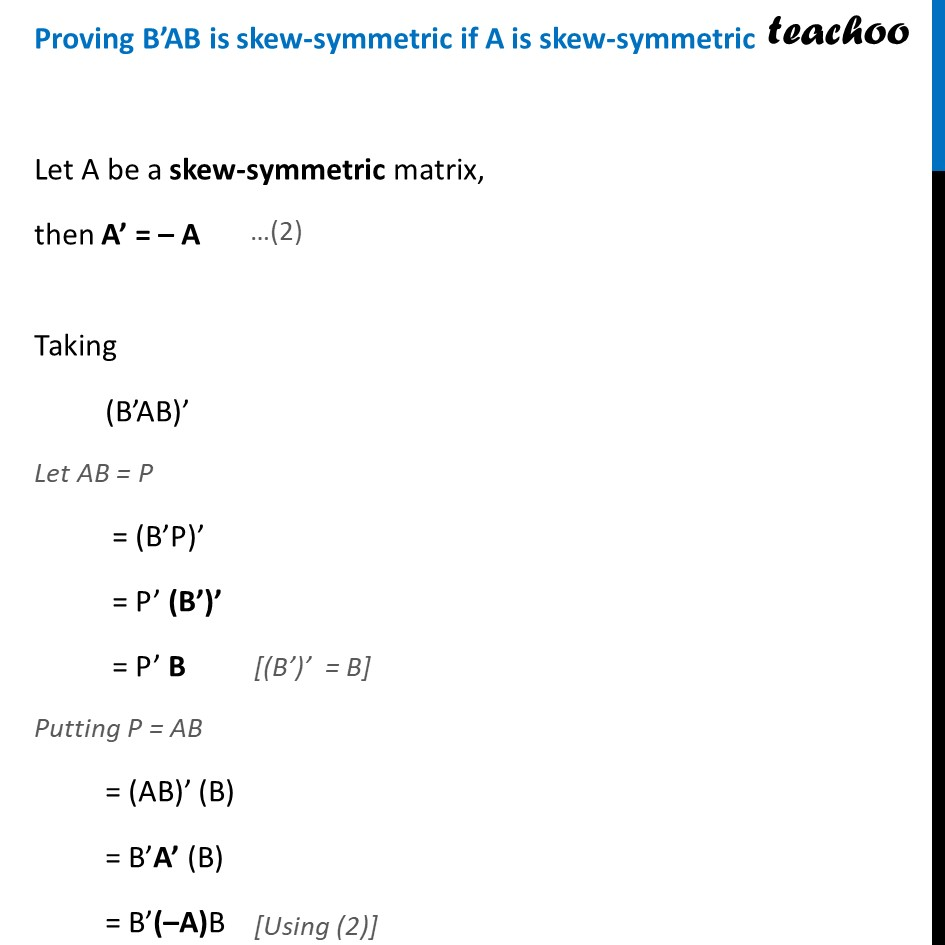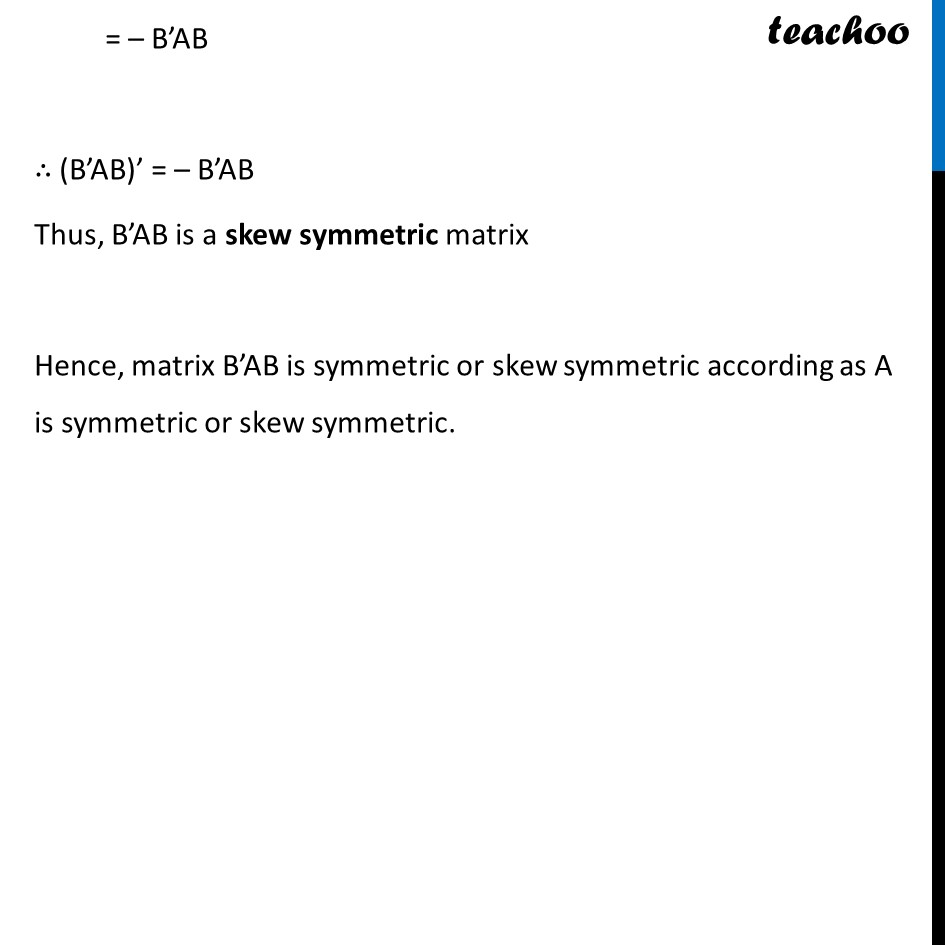Miscellaneous

Chapter 3 Class 12 Matrices
Serial order wiseLearn in your speed, with individual attention - Teachoo Maths 1-on-1 Class

### Transcript

Misc 2 Show that the matrix B’AB is symmetric or skew symmetric according as A is symmetric or skew symmetric. We need to prove B’AB is symmetric if A is symmetric and B’AB is skew symmetric if A is skew symmetric Proving B’AB is symmetric if A is symmetric Let A be a symmetric matrix, then A’ = A Taking (B’AB)’ Let AB = P = (B’P)’ = P’ (B’)’ = P’ B Putting P = AB = (AB)’ (B) = B’A’ (B) = B’AB ∴ (B’AB)’ = B’AB Thus, B’AB is a symmetric matrix Proving B’AB is skew-symmetric if A is skew-symmetric Let A be a skew-symmetric matrix, then A’ = – A Taking (B’AB)’ Let AB = P = (B’P)’ = P’ (B’)’ = P’ B Putting P = AB = (AB)’ (B) = B’A’ (B) = B’(–A)B = – B’AB ∴ (B’AB)’ = – B’AB Thus, B’AB is a skew symmetric matrix Hence, matrix B’AB is symmetric or skew symmetric according as A is symmetric or skew symmetric.